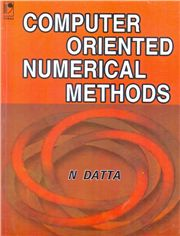# Computer Oriented Numerical Methods, 1/e N Datta-S.CHAND

315.00 285.00

Numerical Methods and errors
• Interpolation
• Numerical Differentiation
• Numerical Integration
• Solution of Algebraic and Transcendental Equations
• Numerical Solution of a system of Linear Equations
• Numerical Solution of Ordinary differential equations
• Curve fitting
• Numerical Solution of problems associated with Partial Differential Equations •Appendix – Programs